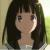# 什么时候double精度出现问题，可以用BigDecimal代替？

ylchen.xd 发布于 2012/12/16 00:11

BigDecimal：

```import java.math.*;;
public class BounceBall
{
private static final int DEF_DIV_SCALE = 10;
public static void main(String[] args)
{
double h = 100.0;
double sum = h;
byte n = 10;
for(int i = 0; i < n; i ++)
{
h = div(h,2);
sum += 2*h;
}
System.out.println("h = " + h + ",sum = " + sum);
}
public static double div(double d1 , double d2)
{
BigDecimal b1 = BigDecimal.valueOf(d1);
BigDecimal b2 = BigDecimal.valueOf(d2);
return b1.divide(b2 , DEF_DIV_SCALE ,
BigDecimal.ROUND_HALF_UP).doubleValue();
}
}```
double：

```public class BounceTest
{
public static void main(String[] args)
{
double h = 100.0;
double sum = h;
byte n = 10;
for(int i = 0; i < n; i ++)
{
h = h/2;
sum += 2*h;
}
System.out.println("h = " + h + ",sum = " + sum);
}
}```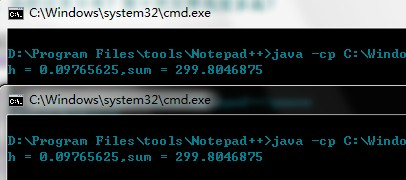0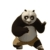```public static void main(String[] args) {
double d=4.0;
System.out.println(foo(d,10));
System.out.println(foo(d,50));
System.out.println(foo(d,53));
}

static double foo(double d, int iter) {
for (int i = 0; i < iter; i++) {
d = Math.sqrt(d);
}
for (int j = 0; j < iter; j++) {
d = d*d;
}
return d;
}```

Out：

4.000000000000094
3.490342924893666
1.0

```	public static void main(String a[]) {
System.out.println( Math.pow(0.2, 1000) );

BigDecimal num=new BigDecimal("0.2");
System.out.println(num.pow(1000));
}```

0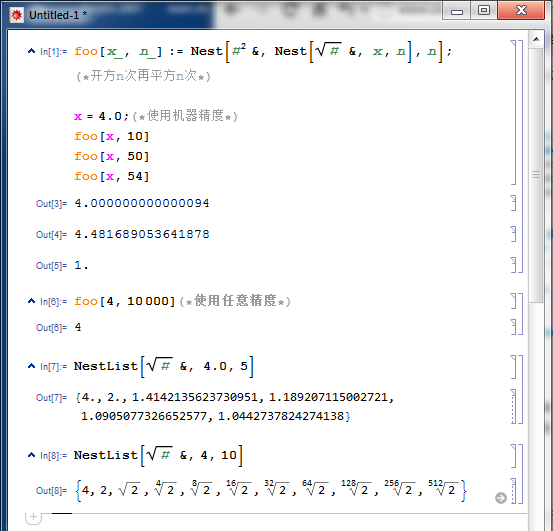0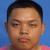1.二进制,乘除2一般不会造成精度损失

2.你的调用深度还不够

3.最重要的是,不损失精度要求一直用BigDecimal,你一会用一会又转回double,再好的精度也损失完了

4.double的精度范围是16位十进制有效数字

0